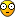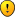## Defi sensors linearisation

jura11
Posts: 22
Joined: Sun Apr 10, 2011 10:30 am

### Defi sensors linearisation

Hi guys

Not sure guys if someone don't have Defi pressure sensor linearisation?
We are wired today Defi Oil and fuel pressure sensor linearisation,we are used only white signal wire and separate black and red we are wired to cigarette lighter or do we should wired this to the Syvecs ?

Please guys if you do have please if you could post them

Thanks,Jura
jura11
Posts: 22
Joined: Sun Apr 10, 2011 10:30 am

### Re: Defi sensors linearisation

I've just realize when I post this,what we are done(I've re-read old emails from Tim,when we are bought the sensors first time and then I realize what we are done)I'm bit mad on myself,just due we shouldn't use 12v for powering the sensor,there is for reason 5vBut sensor survived this and reads like should
But still if you guys have Defi sensors linearisation I would be very appreciated

Thanks,Jura
stevieturbo
Posts: 1016
Joined: Sun Dec 07, 2008 2:04 pm

### Re: Defi sensors linearisation

Handiest way is get a Mityvac ( one that lets you apply pressure too ) or a compressor/regulator and apply pressure to the sensor and monitor voltage on the ecu.

That way you know for sure it's calibrated correctly.
CT9A
Posts: 52
Joined: Thu Mar 07, 2013 11:55 pm

### Re: Defi sensors linearisation

Can you share the defi oil pressure linearisation?
D12SDL
Posts: 5
Joined: Sat Feb 24, 2018 10:01 am

### Re: Defi sensors linearisation

So are you taking the signal feed from your defi sensor and plugging it into the syvecs for the reading ???
Can you share what colour wire you have used for the signal to syvecs please
Posts: 26
Joined: Wed Jan 29, 2014 12:23 am

### Re: Defi sensors linearisation

CT9A wrote: Fri Jan 12, 2018 1:14 pm Can you share the defi oil pressure linearisation?
Defi Pressure Sensor

0psi=.580v
5psi=.753v
10psi=.837v
15psi=.978v
20psi=1.112v
25psi=1.250v
30psi=1.392v
35psi=1.525v
40psi=1.670v
45psi=1.811v
50psi=1.954v
55psi=2.095v
60psi=2.239v
65psi=2.372v
70psi=2.504v
75psi=2.647v
80psi=2.780v
85psi=2.926v
90psi=3.063v
95psi=3.200v
100psi=3.326v
105psi=3.454v
110psi=3.596v
115psi=3.739v
120psi=3.882v
125psi=4.000v
130psi=4.150v
135psi=4.280v
140psi=4.420v

Defi Temperature Sensor

DegC Voltage
25 = 4.548
30 = 4.481
35 = 4.402
40 = 4.3115
45 = 4.212
50 = 4.1045
55 = 3.986
60 = 3.858
65 = 3.726
70 = 3.583
75 = 3.429
80 = 3.2785
85 = 3.119
90 = 2.9625
95 = 2.8105
100 = 2.6595
105 = 2.509
110 = 2.342
115 = 2.2025
120 = 2.0575
125 = 1.9205
130 = 1.775
135 = 1.657
140 = 1.542
145 = 1.435
150 = 1.329
155 = 1.2355
160 = 1.1565
165 = 1.0755
170 = 1.0045
175 = 0.942
180 = 0.8855
185 = 0.8515
190 = 0.814
195 = 0.795
200 = 0.776
PRESSURE - PressureDefimBar.txt